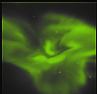Next: Summary Up: The Forward model - Previous: Point spread function   Contents

# Pixel sensitivity

The basic assumption for this analysis is that the camera has an unintensified CCD-detector giving a linear response to both photon intensity and total number of photons. From this assumption it follows that the detected intensity depends linearly on both the total number of photons and the intensity. Thus, provided that no non-linearities are added (Brändström private communication), the output from the pixel with image coordinates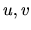is: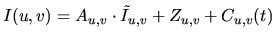(5.18)

where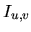is the intensity in pixel,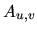is the sensitivity of the pixel,is the number of photons that hit the pixel,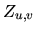are the zero level, and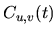the dark current of the pixel.

To calibrate the camera the first step is to make a large series of images with zero time of exposure to get as good an estimate as possible of. The second step is to take a large number of images without any light to estimate the dark current; this has been done with sufficient accuracy for the ALIS cameras.

When the zero level and dark current is determined it is possible to rewrite equation (5.18):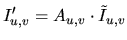(5.19)

where. The third step is to determine the pixel sensitivity matrix. This can be done by taking set of images with different exposure times, which by simple algebra gives the estimate of the sensitivity matrix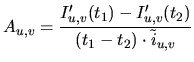(5.20)

whereis the number of photons per second that hit the pixel. The number of photons that hit the pixel has some random variation due to the Poisson characteristics of the emitting source. In addition to the Poisson noise the measured images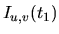and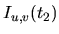contain the internal noise of the camera. Even with an ideal detector, without internal noise, there will be a stochastic variance which is at least as large as the square root of the measured signal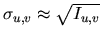. From equation (5.20) it follows that the variance of the sensitivity estimate is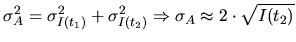(5.21)

If the requirement on the accuracy of the sensitivity estimate is a relative error of, it is perfectly straightforward to derive the necessary condition on the total measured intensity needed: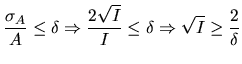(5.22)

This infers that if the accuracy requirements on the sensitivity is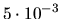the needed total intensities in the pixel,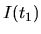andis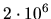counts. With images withcounts it is necessary to take the average of some 50 images to get the necessary accuracy. For ALIS, however, there is as yet no estimate of the sensitivity matrix with the required accuracy. However, there exists relative sensitivity estimates that give the relative average sensitivity of the different cameras.Next: Summary Up: The Forward model - Previous: Point spread function   Contents

copyright Björn Gustavsson 2000-10-24